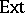##### Actions

Let $R$ be an associative ring with unit and let $N$ be a (unitary right $R$-) module (cf. also Associative rings and algebras; Module). Then $N$ is a Whitehead test module for projectivity (or a p-test module) if for each module $M$, $\operatorname { Ext } _ { R } ^ { 1 } ( M , N ) = 0$ implies $M$ is projective (cf. also Projective module). Dually, $N$ is a Whitehead test module for injectivity (or an i-test module) if for each module $M$, $\operatorname { Ext } _ { R } ^ { 1 } ( N , M ) = 0$ implies $M$ is injective (cf. also Injective module). So, Whitehead test modules make it possible to test for projectivity (injectivity) of a module by computing a single-group.
By Baer's criterion, for any ring $R$ there is a proper class of i-test modules. Dually, for any right-perfect ring (cf. also Perfect ring) there is a proper class of p-test modules. If $R$ is not right perfect, then it is consistent with ZFC (cf. Set theory; Zermelo axiom) that there are no p-test modules, [a2], [a8].
This is related to the structure of Whitehead modules ($M$ is a Whitehead module if $\operatorname { Ext } _ { R } ^ { 1 } ( M , R ) = 0$, [a1]). If $R$ is a right-hereditary ring, then there is a cyclic p-test module if and only if $R$ is p-test if and only if every Whitehead module is projective. The validity of the latter for $R = \mathbf{Z}$ is the famous Whitehead problem, whose independence of ZFC was proved by S. Shelah [a5], [a6], and whose combinatorial equivalent was identified in [a3]. If $R$ is right hereditary but not right perfect, then it is consistent with ZFC that there is a proper class of p-test modules [a8].
Let $\kappa$ be a cardinal number. Then $R$ is $\kappa$-saturated if each non-projective $\leq \kappa$-generated module is an i-test module. If $R$ is $\lambda$-saturated for all cardinal numbers $\lambda$, then $R$ is called a fully saturated ring [a7]. There exist various non-Artinian $n$-saturated rings for $n < \aleph_0$, but all $\aleph_{0}$-saturated rings are Artinian (cf. also Artinian ring). Moreover, all right-hereditary $\aleph_{0}$-saturated rings are fully saturated, and their class coincides with the class of rings $S$, $T$, or $S \boxplus T$, where $S$ is completely reducible and $T$ is Morita equivalent (cf. Morita equivalence) to the upper triangular $( 2 \times 2 )$-matrix ring over a skew-field [a4] (cf. also Matrix ring; Ring with division; Division algebra)